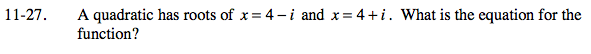Home > PC > Chapter 11 > Lesson 11.1.2 > Problem11-27

11-27.

A quadratic has roots of x = 4 − i and x = 4 + i. What is the equation for the function? Homework Help ✎y = (x − (4 − i))(x − (4 + i))